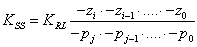﻿ 20-sim webhelp > Toolboxes > Frequency Domain Toolbox > Linear System Editor > Editor > gains

# gains

Given the transfer function: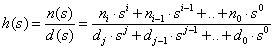the Steady State Gain is defined as: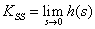Note that the steady state gain can be zero or infinite depending on the element values of the numerator and denominator!

## Root Locus Gain

Given an system described by the transfer function:This transfer function can be rewritten in pole zero notation with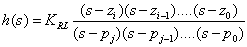where pi .. p1 are the poles and zi .. z1 are the zeros of the system. The gain KRL is known as the Root Locus Gain. Note that it can easily be derived from the transfer function as: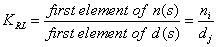## System Gain

Given the transfer function:If n0 and d0 are unequal to zero, this transfer function can be rewritten in pole zero notation with: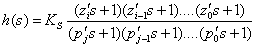The gain KS is known as the System Gain. If n0 is zero and n1 is nonzero an equivalent notation can be found with an extra s multiplied: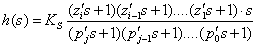If more numerator element are zero, extra multiplications with s are added. The same goes for denominator elements equal to zero. In general the System Gain can be derived from the transfer function as: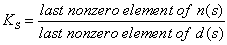## Relating the Gains

The elements of the System Gain are related to the poles and zeros of the Root Locus Gain as: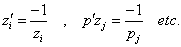If z0 and p0 are unequal to zero, the following equation holds: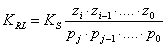The Root Locus Gain and the Steady State Gain are related as: